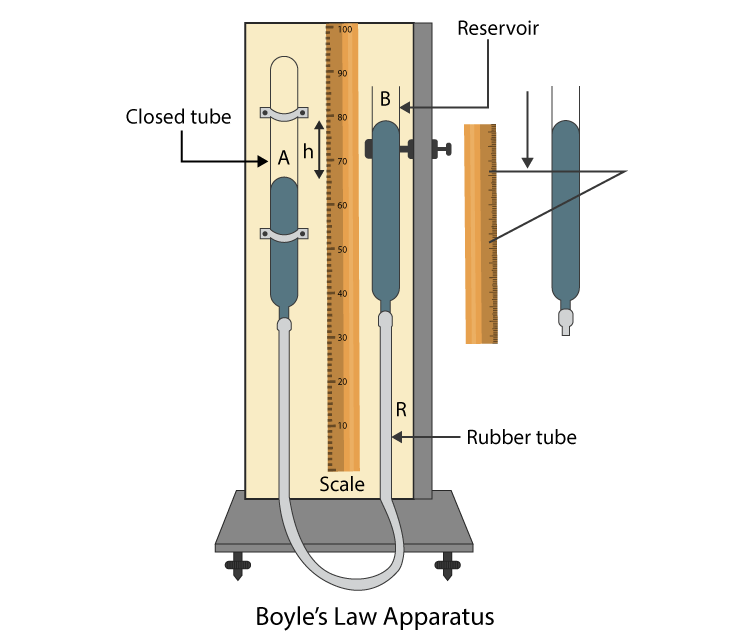# To Study The Variation In Volume With Pressure For A Sample When The Temperature Is Constant By Plotting Graphs Between P And V

Boyle’s law apparatus is used to demonstrate Boyle’s law. To understand how this apparatus is used, below is the experiment explaining how to study the variation in volume with pressure for a sample of air at a constant temperature. The relation between P and V and P and 1/V is studied by plotting graphs.

Aim

To study the variation in volume with pressure for a sample of air at constant temperature by plotting graphs between P and V, and between P and 1/V.

Materials Required

1. Boyle’s law apparatus
2. Plumb line
3. A pair of set-squares
4. A thermometer
5. Fortin’s barometer

Theory

### What is Boyle’s law?

Boyle’s law states that the pressure (P) of an enclosed gas is inversely proportional to its volume (V) and the temperature (T) of the gas is constant.

For an enclosed gas, at a constant temperature (T):

P ∝ 1/V

PV = constant

DiagramProcedure

1. The apparatus is set on a wooden board vertically with the help of levelling screws in the heavy metallic base. Plumb line is used for testing of the apparatus.
2. The upper end of the graduated tube A should be closed as it has air enclosed in it.
3. The mercury level in tube B should be equal to the air level in tube A. Air pressure in tube A is equal to the atmospheric pressure on the mercury in tube B.
4. The atmospheric pressure should be noted from Fortin’s barometer.
5. The temperature should be noted down from the thermometer attached to the barometer.
6. The mercury level in tube A and B will be the same and can be measured using scale S.
7. Note the volume of enclosed gas in graduated tube A.
8. The volume of enclosed air reduces as tube B is moved up by 2cm. Mercury level in tube A also reduces.
9. As the pressure of air increases in tube A, the mercury level will be lower than tube B.
10. Using set-squares the mercury level in tube B is noted which is against the vertical scale.
11. For two more readings, repeat steps 8,9 and 10 by raising tube B by 2cm.
12. To bring the mercury level in the same horizontal line as in tube A, lower the tube B. Check the reading by step 6.
13. By moving tube B downward by 2cm, the mercury level in tube A will reduce with an increase in the volume of enclosed air. Note the increased volume.
14. The pressure of air in tube A decreases and the mercury level increases more than tube B. Repeat step 10.
15. Repeat steps 13 and 14 two more times by lowering the tube B by 2cm.
16. Repeat steps 4 and 5.
17. Record the observations in tabular form.

Observations

Initial atmospheric pressure, P1 = …….cm of Hg

Final atmospheric pressure, P2 = …….cm of Hg

Mean atmospheric pressure,

$$\begin{array}{l}P_{0}=\frac{P_{1}+P_{2}}{2}=75.0\;cm\;of\;Hg\end{array}$$

Temperature almost remained the same during observations and T = ………℃

Table for mercury levels in the tube A and B

 Position of Hg level Pressure difference (p) (cm) Tube A-Tube B Pressure of air P = P0+p (cm of Hg) Volume of air V (cm3) 1/V (cm-1) PV In tube A (cm) In tube B (cm)

Calculations

Through table:

1. Find the difference of Hg levels in tube A and tube B to find pressure difference (p).
2. Add atmospheric pressure P0 (75 cm of Hg) to pressure difference p to find total pressure P = P0+p of air in the tube A.
3. Write volume V of air enclosed in tube A.
4. Write the value of 1/V and PV in the respective columns.

Through graph

1. Draw a graph between P and V taking P on x-axis and V on the y-axis. The graph will be a hyperbola.
2. Draw another graph between P and 1/V taking 1/V on x-axis and P on the y-axis. The graph will be a straight line with a positive slope.

Result

1. PV is constant and is according to Boyle’s law.
2. P-V graph is a hyperbola and satisfies the relation PV = constant.
3. P-1/V graph is a straight line with a positive slope and satisfies the relation P∝ 1/V.

Precautions

1. Plotting of graphs must be done with at most care.
2. The wooden board must be vertical.
3. To note the levels of mercury must be taken with set-squares.
4. Atmospheric pressure must be calculated at the beginning and the end of the experiment. Mean of atmospheric pressure must be used in the calculation.
5. Pure and dry air should be used in tube A.

Sources Of Error

1. Pure and dry air is not used in tube A.
2. The base might not be broad and the stability of the apparatus may get disturbed.

Viva Questions

Q1. What is atmospheric pressure?

Ans: Atmospheric pressure is defined as the pressure on the earth surface due to the air column. Pa = 1.013×10-5 Nm-2

Q2. What is the ideal gas equation?

Ans: Following is the ideal gas equation:

 PV = nRT

Q3. For which gas Boyle’s law is verified in the experiment?

Ans: For air Boyle’s law is verified in the experiment.

Q4. Does Boyle’s law hold good under all the conditions?

Ans: No, Boyle’s law holds good when the temperature is high and pressure is low.

Q5. When the temperature is constant, what is the nature of the graph between pressure and volume?

Ans: Hyperbola.